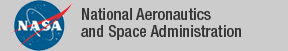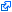### Top File of PVS orders Library

```
top: THEORY
%----------------------------------------------------------------------------
%
%  Orders Library
%  --------------
%
%  Version 1.0    Extracted From sets_aux library on 4/21/05
%  Version 1.1    Added finite_below, similarity, and similarity_props
%
%  Authors:
%      Bruno Dutertre  SRI International
%      Jerry James        University of Kansas
%      Alfons Geser     National Institute For Aerospace
%----------------------------------------------------------------------------
BEGIN

%---- Alfons Geser theories on order
IMPORTING
bounded_integers,         % nonempty, above/below bounded => greatest/least
bounded_orders,           % definitions of lub, glb, (complete) lattice
bounded_sets,             % judgements about boundedness properties of sets
closure_ops,              % reflexive, symmetric, transitive, etc. closure
complementary_lattices,   % lattices with a "complement" function
complementary_orders,     % ordered sets with a "complement" function
complete_lattices,        % every set is tightly bounded
complete_lower_semilattices, % every set is greatest bounded below
complete_upper_semilattices, % every set is least bounded above
finite_orders,            % properties of an order on a finite type
finite_pointwise_orders,  % orders on functions with a finite domain
finite_total_orders,      % total orders on a finite type
fixed_points,             % fixed points characterized by prefixed points
integer_enumerations,     % infinite below bounded set of ints => enumerable
lattices,                 % operations that preserve tight-boundedness
lower_semilattices,       % definition of binary glb function
minmax_orders,            % minimal, maximal, least, greatest elements
monotone_sequences,       % infinite ascending/descending sequences
new_mucalculus_prop,      % a simulation of fixedpoints@mucalculus_prop
non_empty_bounded_sets,   % nonempty sets of reals bounded above/below
pointwise_orders,         % lifting an order to functions
sets_complete_lattices,   % sets ordered by "subset?" form a complete lattice
total_lattices,           % a lattice defined by a total order
upper_semilattices,       % definition of binary lub function
well_foundedness          % well-foundedness = no infinite descending seq.

%---- Jerry James theories on order
IMPORTING
bounded_nats,             % all nonempty sets of nats have a least element
chain,                    % totally ordered subsets of a poset
chain_chain,              % chains of chains in inclusion order
converse_zorn,            % lower bound on all chains => min. element exists
finite_below,             % finite set = monotonic bijection with below[N]
isomorphism,              % isomorphisms between ordered sets
isomorphism_equivalence,  % automorphisms and equivalence classes thereof
isomorphism_symmetric,    % the isomorphic? relation is symmetric
isomorphism_transitive,   % the isomorphic? relation is transitive
kuratowski,               % there exists a maximal chain in any set
monotone_functions,       % (non)in/decreasing functions on ordered sets
numbers_infinite,         % the nats, ints, rats, and reals are infinite
order_strength,           % strengthenings and weakenings of orders
ordered_int,              % ordered subsets of the integers
ordered_nat,              % ordered subsets of the natural numbers
ordered_subset,           % prefix, suffix, upto, below, upfrom, etc.
range,                    % open and closed ranges of numbers
range_real,               % the range theory, tailored to ranges of reals
set_antisymmetric,        % injective maps both ways => bijection exists
set_dichotomous,          % injective map exists between any 2 sets
similarity,               % similar ordered sets: order-preserving bijection
similarity_props,         % least, greatest, and all finite sets are similar
subset_chain,             % chains of sets in inclusion order
well_nat,                 % well-ordered relations on sets of nats
well_ordered_finite,      % linear order + finite below sets = well-ordered
well_ordered_props,       % some properties of well-ordered sets
well_ordered_traversal,   % first, last, next, & prev for well-ordered sets
well_ordering,            % every set has a well-ordering relation
zorn                      % upper bound on all chains => max. element exists

%---- Alfons Geser prelude-style theories
IMPORTING
booleans_are_finite,      % the booleans are a finite type
finite_types,             % if T is finite, then every set of T is finite
function_image_extra,     % Two more rewrite rules for function_image
indexed_sets_extra,       % IUnion and IIntersection are monotone
infinite_pigeonhole,      % [infinite_domain -> finite_range] enumerates
% some range element infinitely often
relation_iterate,         % R o R o ... o R  n times.
relations_extra,          % rewrite rules and judgements for binary relations
skolemization,            % how to eliminate inner quantifiers

%--- New Content From David Lester, Manchester

directed_orders,        % New theories for orders library
bounded_order_props,    % Properties of bounded orders
directed_order_props,   % Properties of directed orders
partial_order_props,    % Properties of partial orders
lift_props,             % Extras for the lift datatype
lifted_orders,          % Induced properties of lifted orders
partial_order_lift,
sum_orders,             % Induced properties of union orders
product_orders          % Induced properties of product orders

%------- Bruno Dutertre
IMPORTING
mucalculus_prop           % originally in library fixedpoints

END top
```
The tagidentifies links that are outside the NASA domain